##### The Japanese Bonsai specialist
Direct order Contact Help / Services Newsletter# Hosta ben vernooij

Hosta ben vernooij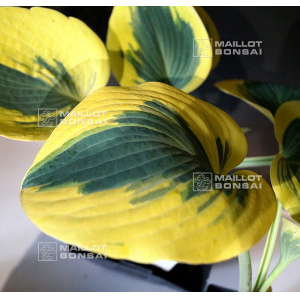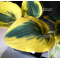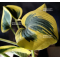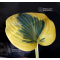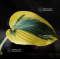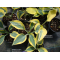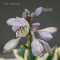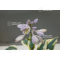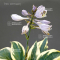ref. : 11037

12,00

Available quantity : 15Order

###### Description

Coming from a hybridization of Hosta First frost.

Similar to Autumn frost and First frost.

Dimensions of the leaves : 18x13 cm.

The leaves are blue/green blue at the middle and the sides of the leaves are large and yellow / yellow ochre.

The flowering color of this variety is lavender and the flowers appear in summer.

Big rhizome delivered in a 1 liter plastic pot.

#leaves 3.6 #hosta 3.5 #frost 3.5 #vernooij 2.8 #yellow 2.6 #hostas 2.6 #first 2.5 #about 2.5 #blue 2.4 #hybridization 2.3

###### Technical description
 height 30-50 cm

Formule
(( ROUND((CHAR_LENGTH(b.article_nom)-CHAR_LENGTH(REPLACE(b.article_nom, 'leaves', '')))/LENGTH('leaves')) + ROUND((CHAR_LENGTH(b.article_description)-CHAR_LENGTH(REPLACE(b.article_description, 'leaves', '')))/LENGTH('leaves')) ) * 3.6) + (( ROUND((CHAR_LENGTH(b.article_nom)-CHAR_LENGTH(REPLACE(b.article_nom, 'hosta', '')))/LENGTH('hosta')) + ROUND((CHAR_LENGTH(b.article_description)-CHAR_LENGTH(REPLACE(b.article_description, 'hosta', '')))/LENGTH('hosta')) ) * 3.5) + (( ROUND((CHAR_LENGTH(b.article_nom)-CHAR_LENGTH(REPLACE(b.article_nom, 'frost', '')))/LENGTH('frost')) + ROUND((CHAR_LENGTH(b.article_description)-CHAR_LENGTH(REPLACE(b.article_description, 'frost', '')))/LENGTH('frost')) ) * 3.5) + (( ROUND((CHAR_LENGTH(b.article_nom)-CHAR_LENGTH(REPLACE(b.article_nom, 'vernooij', '')))/LENGTH('vernooij')) + ROUND((CHAR_LENGTH(b.article_description)-CHAR_LENGTH(REPLACE(b.article_description, 'vernooij', '')))/LENGTH('vernooij')) ) * 2.8) + (( ROUND((CHAR_LENGTH(b.article_nom)-CHAR_LENGTH(REPLACE(b.article_nom, 'yellow', '')))/LENGTH('yellow')) + ROUND((CHAR_LENGTH(b.article_description)-CHAR_LENGTH(REPLACE(b.article_description, 'yellow', '')))/LENGTH('yellow')) ) * 2.6) + (( ROUND((CHAR_LENGTH(b.article_nom)-CHAR_LENGTH(REPLACE(b.article_nom, 'first', '')))/LENGTH('first')) + ROUND((CHAR_LENGTH(b.article_description)-CHAR_LENGTH(REPLACE(b.article_description, 'first', '')))/LENGTH('first')) ) * 2.5) + (( ROUND((CHAR_LENGTH(b.article_nom)-CHAR_LENGTH(REPLACE(b.article_nom, 'about', '')))/LENGTH('about')) + ROUND((CHAR_LENGTH(b.article_description)-CHAR_LENGTH(REPLACE(b.article_description, 'about', '')))/LENGTH('about')) ) * 2.5) + (( ROUND((CHAR_LENGTH(b.article_nom)-CHAR_LENGTH(REPLACE(b.article_nom, 'blue', '')))/LENGTH('blue')) + ROUND((CHAR_LENGTH(b.article_description)-CHAR_LENGTH(REPLACE(b.article_description, 'blue', '')))/LENGTH('blue')) ) * 2.4) + (( ROUND((CHAR_LENGTH(b.article_nom)-CHAR_LENGTH(REPLACE(b.article_nom, 'hybridization', '')))/LENGTH('hybridization')) + ROUND((CHAR_LENGTH(b.article_description)-CHAR_LENGTH(REPLACE(b.article_description, 'hybridization', '')))/LENGTH('hybridization')) ) * 2.3) + (( ROUND((CHAR_LENGTH(b.article_nom)-CHAR_LENGTH(REPLACE(b.article_nom, 'dimensions', '')))/LENGTH('dimensions')) + ROUND((CHAR_LENGTH(b.article_description)-CHAR_LENGTH(REPLACE(b.article_description, 'dimensions', '')))/LENGTH('dimensions')) ) * 2)

## Secure payment## Delivery

Our logistic partners :04 74 55 23 48
Pépinière MAILLOT-BONSAÏ
Le Bois Frazy
01990 RELEVANT - FRANCE
on appointment